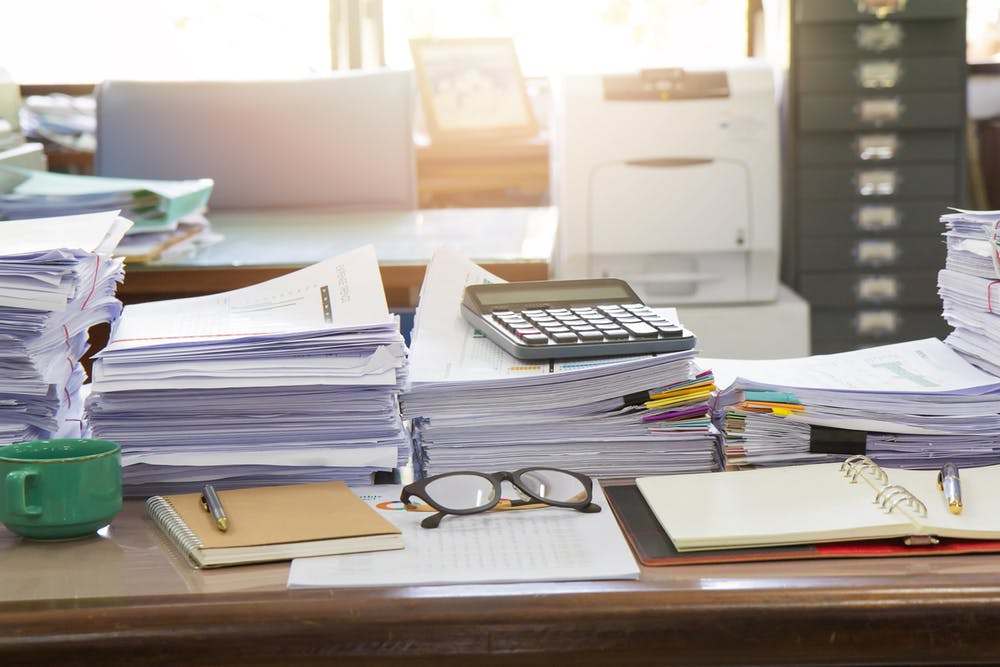# The ‘Arithmetica’ of Diophantus: Cracking Open Centuries-Old Mathematical Puzzles

Mathematicians have known how to solve something called an S-unit equation for several years. However, the process is so convoluted that few can actually use it to tackle their problems.

By Dr. Christopher Rasmussen
Associate Professor of Mathematics
Wesleyan University

In mathematics, no researcher works in true isolation. Even those who work alone use the theorems and methods of their colleagues and predecessors to develop new ideas.

But when a known technique is too difficult to use in practice, mathematicians may neglect important – and otherwise solvable – problems.

Recently, I joined several mathematicians on a project to make one such technique easier to use. We produced a computer package to solve a problem called the “S-unit equation,” with the hope that number theorists of all stripes can more easily attack a wide variety of unsolved problems in mathematics.

## Diophantine equations

In his text “Arithmetica,” the mathematician Diophantus looked at algebraic equations whose solutions are required to be whole numbers. As it happens, these problems have a great deal to do with both number theory and geometry, and mathematicians have been studying them ever since.

Why add this restriction of only whole-number solutions? Sometimes, the reasons are practical; it doesn’t make sense to raise 13.7 sheep or buy -1.66 cars. Additionally, mathematicians are drawn to these problems, now called Diophantine equations. The allure comes from their surprising difficulty, and their ability to reveal fundamental truths about the nature of mathematics.

In fact, mathematicians are often uninterested in the specific solutions to any particular Diophantine problem. But when mathematicians develop new techniques, their power can be demonstrated by settling previously unsolved Diophantine equations.

Andrew Wiles’ proof of Fermat’s Last Theorem is a famous example. Pierre de Fermat claimed in 1637 – in the margin of a copy of “Arithmetica,” no less – to have solved the Diophantine equation xⁿ + yⁿ = zⁿ, but offered no justification. When Wiles proved it over 300 years later, mathematicians immediately took notice. If Wiles had developed a new idea that could solve Fermat, then what else could that idea do? Number theorists raced to understand Wiles’ methods, generalizing them and finding new consequences.

No single method exists that can solve all Diophantine equations. Instead, mathematicians cultivate various techniques, each suited for certain types of Diophantine problems but not others. So mathematicians classify these problems by their features or complexity, much like biologists might classify species by taxonomy.

## Finer classification

This classification produces specialists, as different number theorists specialize in the techniques related to different families of Diophantine problems, such as elliptic curvesbinary forms or Thue-Mahler equations.

Within each family, the finer classification gets customized. Mathematicians develop invariants – certain combinations of the coefficients appearing in the equation – that distinguish different equations in the same family. Computing these invariants for a specific equation is easy. However, the deeper connections to other areas of mathematics involve more ambitious questions, such as: “Are there any elliptic curves with invariant 13?” or “How many binary forms have invariant 27?”

The S-unit equation can be used to solve many of these bigger questions. The S refers to a list of primes, like {2, 3, 7}, related to the particular question. An S-unit is a fraction whose numerator and denominator are formed by multiplying only numbers from the list. So in this case, 3/7 and 14/9 are S-units, but 6/5 is not.

The S-unit equation is deceptively simple to state: Find all pairs of S-units which add to 1. Finding some solutions, like (3/7, 4/7), can be done with pen and paper. But the key word is “all,” and that is what makes the problem difficult, both theoretically and computationally. How can you ever be sure every solution has been found?

In principle, mathematicians have known how to solve the S-unit equation for several years. However, the process is so convoluted that no one could ever actually solve the equation by hand, and few cases have been solved. This is frustrating, because many interesting problems have already been reduced to “just” solving some particular S-unit equation.

## How the solver worksThe process of solving the S-unit equation is so convoluted that few have attempted to do it by hand.Jat306/shutterstock.com

Circumstances are changing, however. Since 2017, six number theorists across North America, myself included, have been building an S-unit equation solver for the open-source mathematics software SageMath. On March 3, we announced the completion of the project. To illustrate its application, we used the software to solve several open Diophantine problems.

The primary difficulty of the S-unit equation is that while only a handful of solutions will exist, there are infinitely many S-units that could be part of a solution. By combining a celebrated theorem of Alan Baker and a delicate algorithmic technique of Benne de Weger, the solver eliminates most S-units from consideration. Even at this point, there may be billions of S-units – or more – left to check; the program now tries to make the final search as efficient as possible.

This approach to the S-unit equation has been known for over 20 years, but has been used only sparingly, because the computations involved are complicated and time-consuming. Previously, if a mathematician encountered an S-unit equation that she wanted to solve, there was no automated way to solve it. She would have to carefully step through the work of Baker, de Weger and others, then write her own computer program to do the computations. Running the program could take hours, days or even weeks for the computations to finish.

Our hope is that the software will help mathematicians solve important problems in number theory and enhance their understanding of the nature, beauty and effectiveness of mathematics.